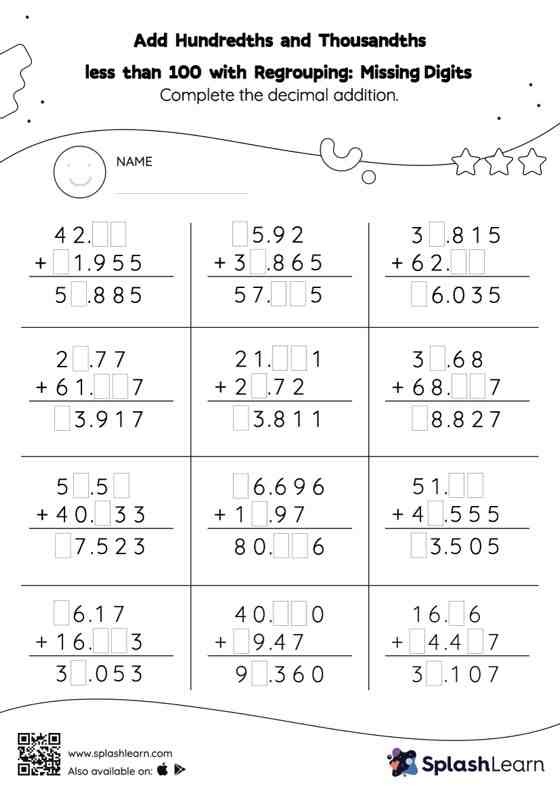# Add Hundredths and Thousandths less than 100 with Regrouping: Missing Digits Worksheet

Home > Add Hundredths and Thousandths less than 100 with Regrouping: Missing DigitsThis worksheet challenges young mathematicians to hone their math skills by solving a set of problems on adding hundredths and thousandths less than 100 with regrouping. While adding decimals, students use the relationship between addition and subtraction to find the missing number. They also need to regroup numbers in add hundredths and thousandths less than 100 with regrouping worksheet utilizing the relationship between ones, tenth, hundredth, thousandth, etc., To get to the result. In this worksheet, students practice solving problems using the column method. This method is especially helpful with problems involving bigger multi-digit numbers as the format provides an easy structure to follow.